# The elasticity of Supply- Meaning, Types and Methods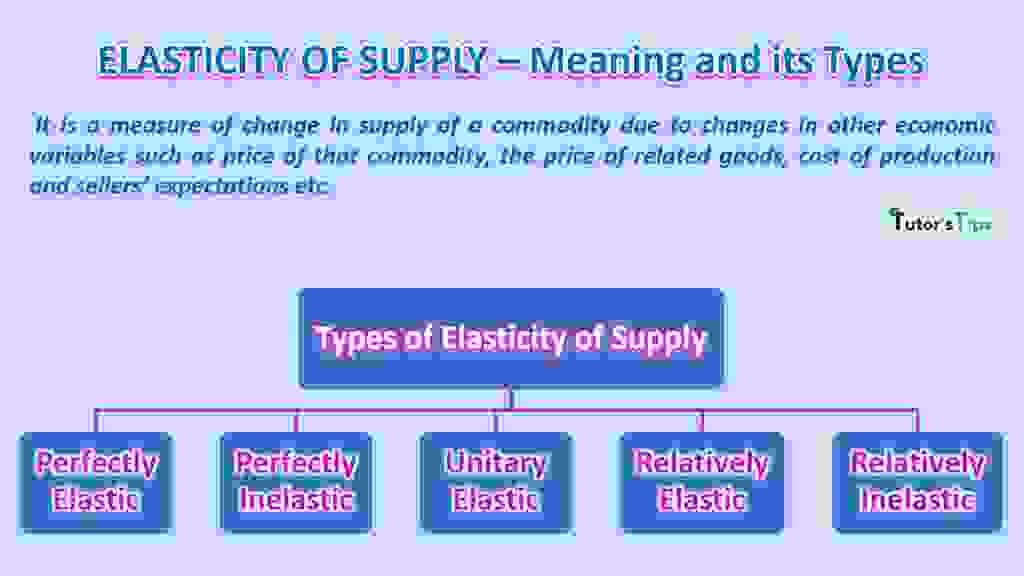The elasticity of supply is an economic term that refers to the sensitivity of supply of a good or service to changes in other economic variables such as the price of goods, price of related goods and sellers’ expectations etc.

## Meaning of Elasticity of Supply :

The elasticity of supply is the responsiveness of quantity supplied of a product to changes in one of the variables on which supply depends. According to basic economic theory, the supply of a commodity increases with a rise in price and decreases with a fall in price.

## Definitions:

According to Bilas,

“Elasticity of Supply is defined as the percentage change in quantity supplied divided by percentage change in price.”

According to Lipsey,

“Elasticity of Supply is the ratio of the percentage change in quantity supplied over the percentage change in price.”

## Price Elasticity of Supply:

The term which measures the rate of quantity demanded due to change in price is known as Price Elasticity of Supply. It measures the relationship between the change in quantity supplied and change in the price of a commodity.

It can be calculated as:

 Price Elasticity of Supply(PES) = Percentage change in quantity supplied Percentage change in price

### Methods to measure the Price Elasticity of Supply:

There are mainly two methods of measuring the price elasticity of supply which are:

1)Proportionate or Percentage Method

2) Geometric Method

These methods can be explained as:

### 1.Proportionate or Percentage Method :

According to this method, the elasticity of supply can be defined as the ratio between ‘percentage change in quantity supplied’ and ‘a percentage change in price’ of the commodity.

Es= Percentage change in quantity supplied/Percentage change in price

Symbolically,

 Es = ΔQ X 100 Q ΔP X 100 P

 Es = ΔQ X P ΔP Q

For Example,

Suppose, a seller offers to sell 500 units of a commodity when its price is Rs.10 per unit, while only 250 units are offered if price reduces to Rs.5 per unit. Then,

 Es = ΔQ X P ΔP Q
 Es = 250-500 X 10 5-10 500
 Es = -250 X 10 -5 500

Es  = 1

### 2. Geometric Method :

Geometrically, the elasticity of supply depends on the origin of the supply curve. Assuming the supply curve to be a straight line and positive sloped, the geometric method measures the elasticity of supply as follows: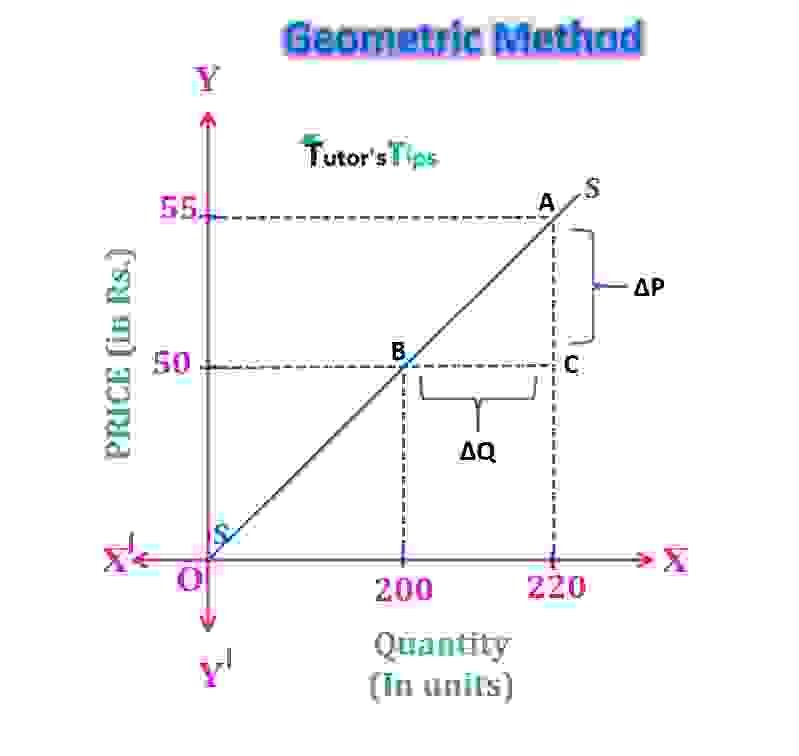In fig,

P= Rs 50 (initial price)

P1= Rs 55 (new price)

Q= 200 units (initial quantity)

Q1= 220 units (new quantity)

ΔP = 55-50 = Rs 5

ΔQ = 220-200 = 20 units

 Es = ΔQ X P ΔP Q

 Es = BC X P AC Q

 Es = 20 X 50 5 200

Es = 1

## Types of Price Elasticity of Supply:

1. Perfectly elastic
2. Perfectly inelastic
3. Unitary elastic
4. Relatively elastic
5. Relatively inelastic

### a)Perfectly Elastic Supply :

The supply is said to be perfectly elastic when a small rise in price would result in supply to become infinite, while a small fall in price would result in a fall in supply to zero. It is also known as infinite elasticity.

This is shown by a straight line supply curve parallel to the horizontal axis.

#### For Example,

Suppose the price of a commodity is Rs.10 and its Supply is 50 units. As the price increases to Rs.15, its supply increases to infinity.In fig, the X-axis shows the quantity and Y-axis shows the price. SS is the supply curve. The initial supply at price P is Q units. When the price is slightly increased, it leads to an increase in supply by large amount i.e. Q1. Thus, it shows perfectly elastic supply.

### b) Perfectly Inelastic Supply :

When the supply doesn’t change with the change in price(whether falling or rising), the supply is said to be perfectly inelastic. It means supply remains constant against any value of price in the market. This is represented by a straight line parallel to the vertical axis.

#### For example,

Suppose the price of a commodity is Rs 10, and supply is 200 units. As the price increases to Rs 20, the Supply remains constant at 200 units. It implies that the supply is perfectly inelastic.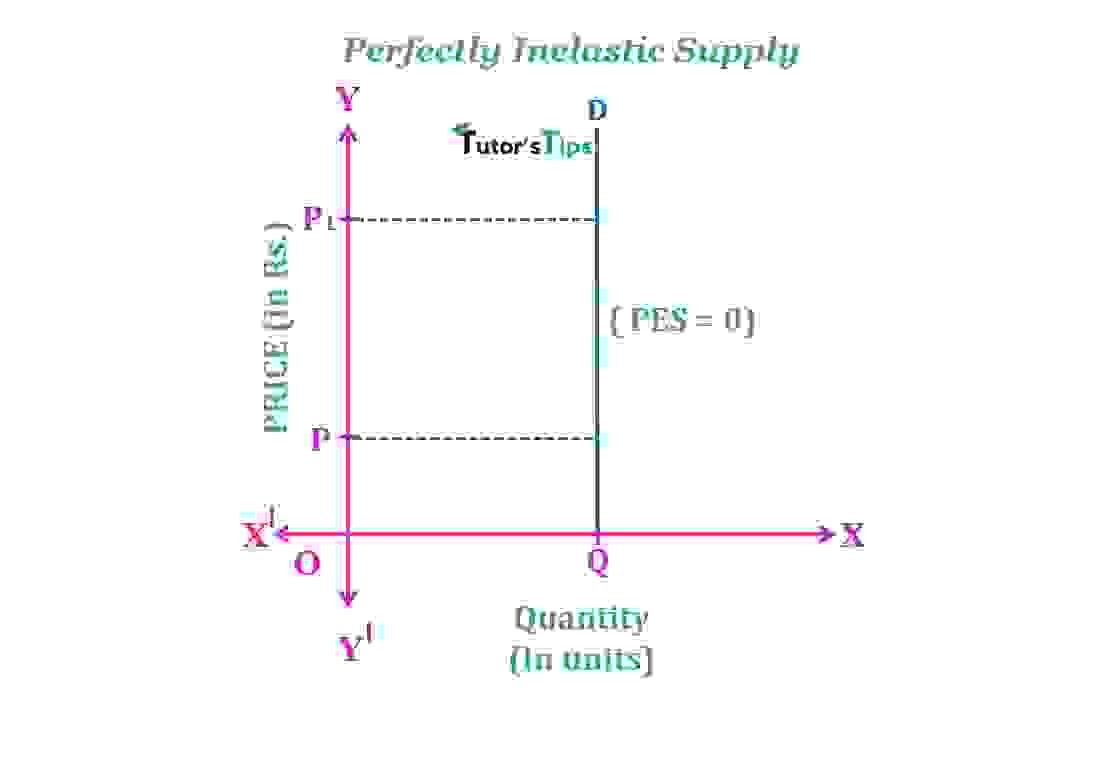In fig, X-axis shows the quantity and Y-axis shows the price.SS is the supply curve. At price P, the quantity supplied is Q units. As the price increases to P1, there is no effect on the quantity supplied. It remains constant at initial quantity Q. Thus, it implies that the supply is perfectly inelastic.

### c) Unitary Elastic Supply:

When the change in the supply of a commodity is in the same ratio as the change in its price, it is known as unitary elastic supply.

#### For Example,

Suppose the price of a commodity is Rs.50 and the quantity supplied in a specific market is 200 units. As the price increases to Rs.55, its supply rises to 220 units. It implies the unitary elastic supply.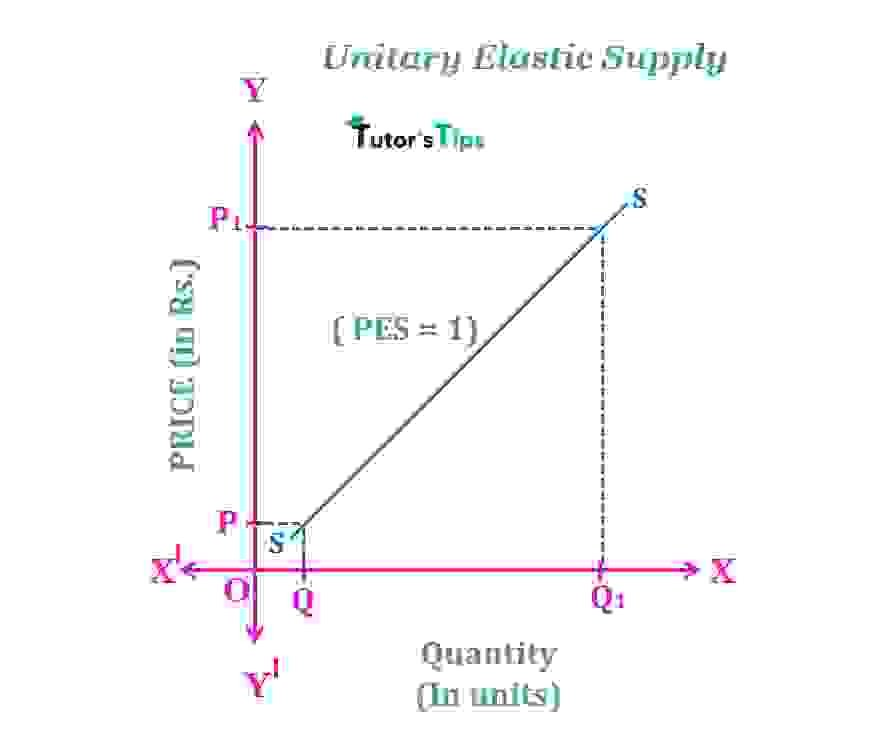In fig, X-axis shows the quantity and Y-axis shows the price. SS is the supply curve. When the price is P, the quantity supplied is Q units. As price increases to P1, the quantity supplied also rises to Q1 with the same proportion. That is why it is known as a unitary elastic supply.

### d) Relatively Elastic Supply :

Relatively Elastic Supply occurs when the proportionate change in supply is greater than proportionate change in price. It means that there will be a greater change in supply due to a small change in price. It is also known as highly elastic supply and more than unitary elastic supply.

#### For Example,

Suppose the price of a commodity is Rs50 and quantity supplied is 200 units. As the price increases to Rs55, its supply increases to 250 units. It implies the supply to be relatively elastic.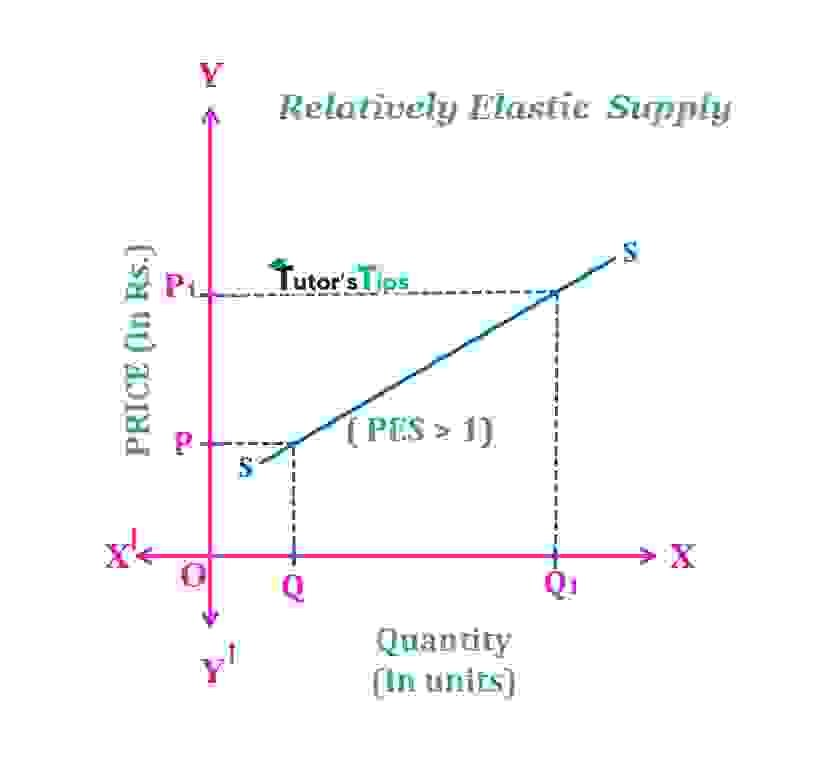In fig, X-axis shows the quantity and Y-axis shows the price. SS is the supply curve. When the price is P, the quantity supplied is Q units. As the price increases to P1, the quantity supplied also increases to Q1 but with more proportion. Thus, it implies a relatively elastic supply.

### e)Relatively Inelastic Supply :

The supply is said to be relatively inelastic when a proportionate change in quantity supplied is less than proportionate change in price. It means that greater change in price leads to a smaller change in quantity supplied.

#### For Example,

Suppose the price of a commodity is Rs50 and quantity supplied is 200 units. As the price increases to Rs70, its supply increases to 220 units. It implies the supply to be relatively inelastic.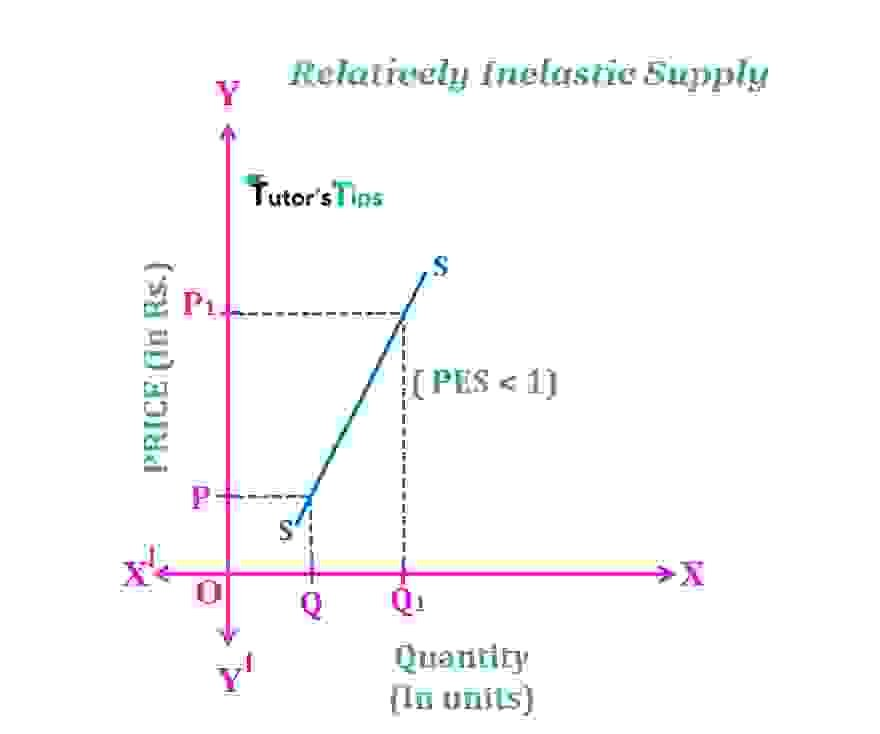In Fig, X-axis shows the quantity and Y-axis shows the price. SS is the supply curve. When the price is P, the quantity supplied is Q units. As the price increases to P1, the supply also increases to Q1 units with less proportion as compared to price. It implies the relatively inelastic supply.

Thanks, Please share with your friends this topic

Comment if you have any question.

References:

Introductory Microeconomics – Class 11 – CBSE (2020-21)